# 起因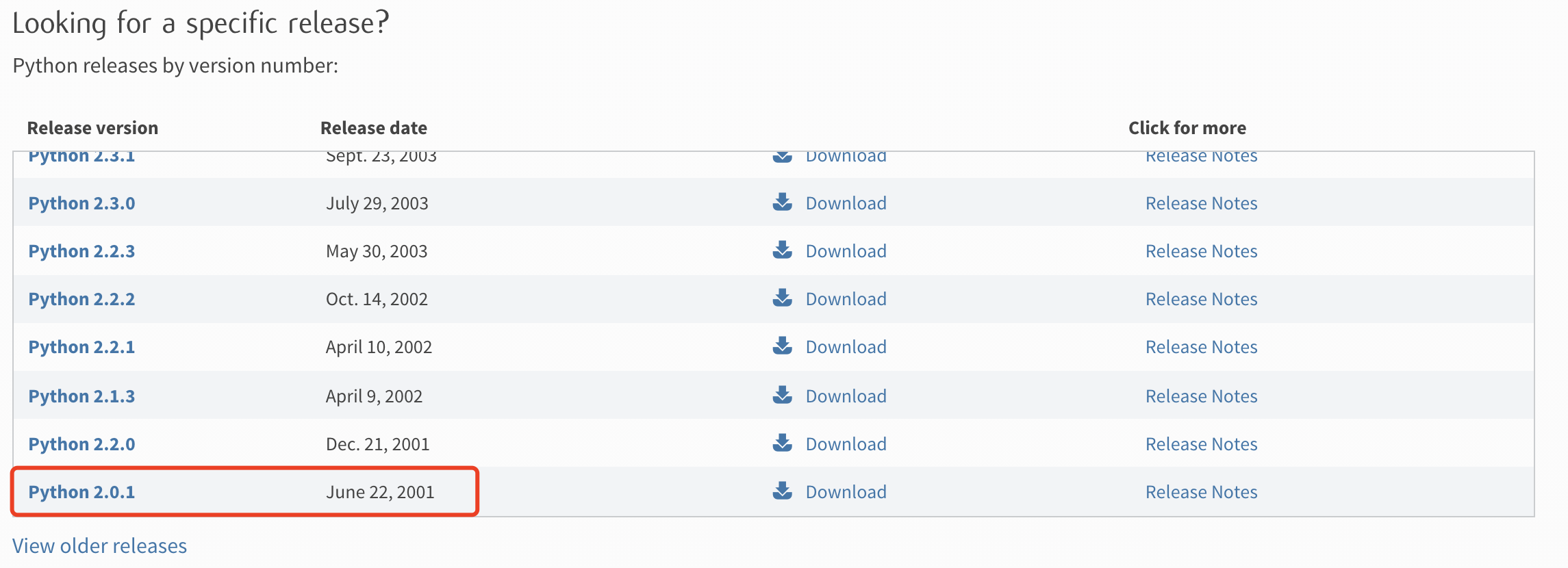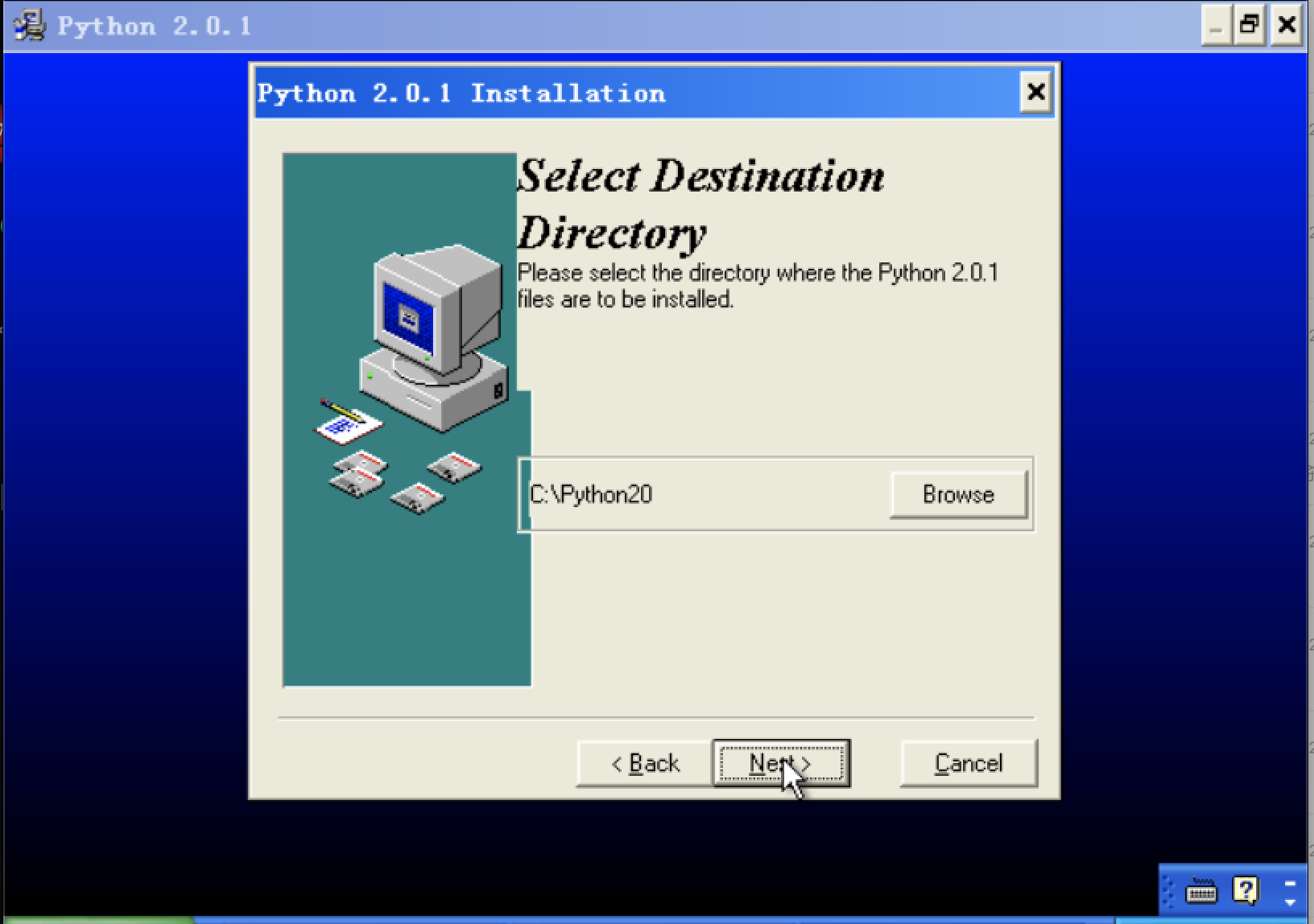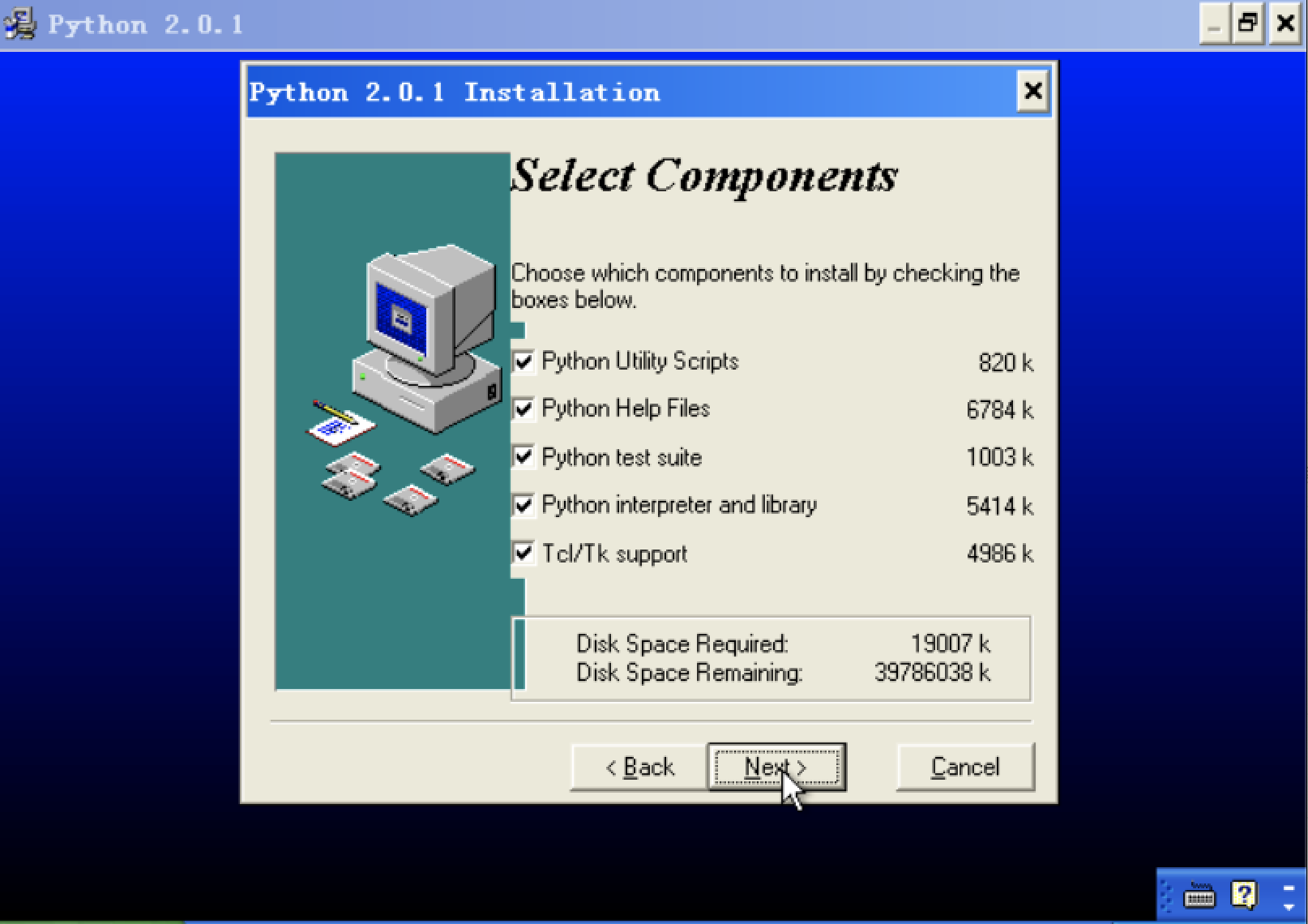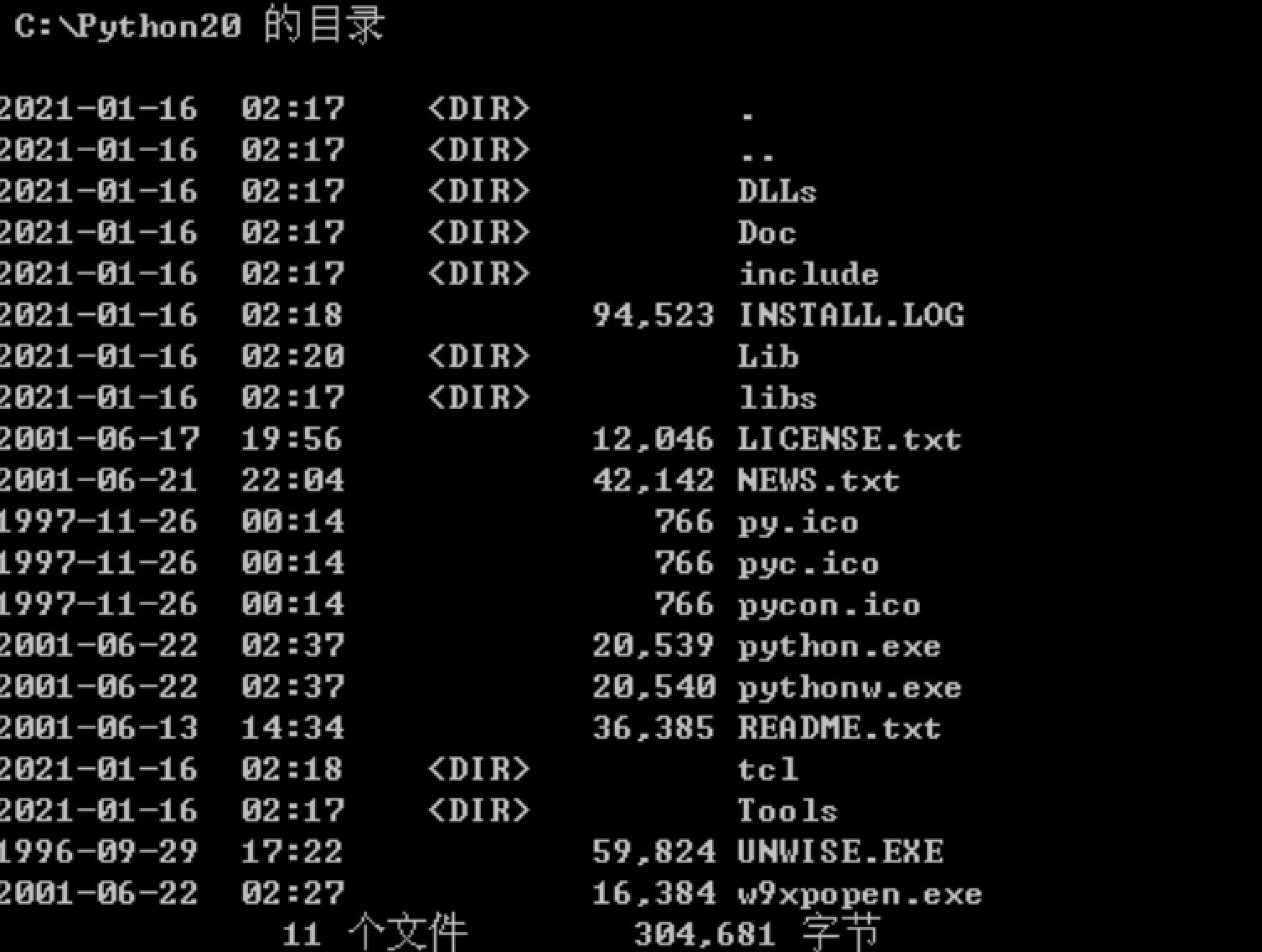x = 2

def re_f():
x = 3
def tmp_f():
print x
tmp_f()

re_f()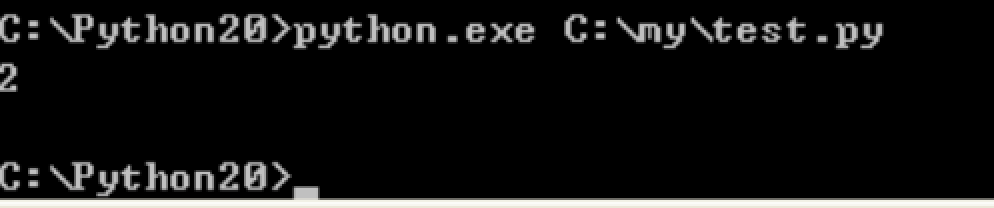??? 什么鬼? 为什么读到了全局变量? 我还特地有到Python 3.0的环境中跑了一遍, 发现结果确实是3啊. 不懂就要问, 于是我开始搜寻各种资料, 发现这设计到了变量的作用域.

# 回顾历史

### 变量回写

function test(){
// 这里用到了变量 i, 那就先把原来的值记下来
$old_i =$i;
// 然后就可以随意对变量 i 修改了
// 返回时将变量改回去
$i =$old_i;
return;
}

$i = 1; function fun_1(){$old_i = $i;$i = 2;
fun_2();
$i =$old_i;
return;
}

### 动态作用域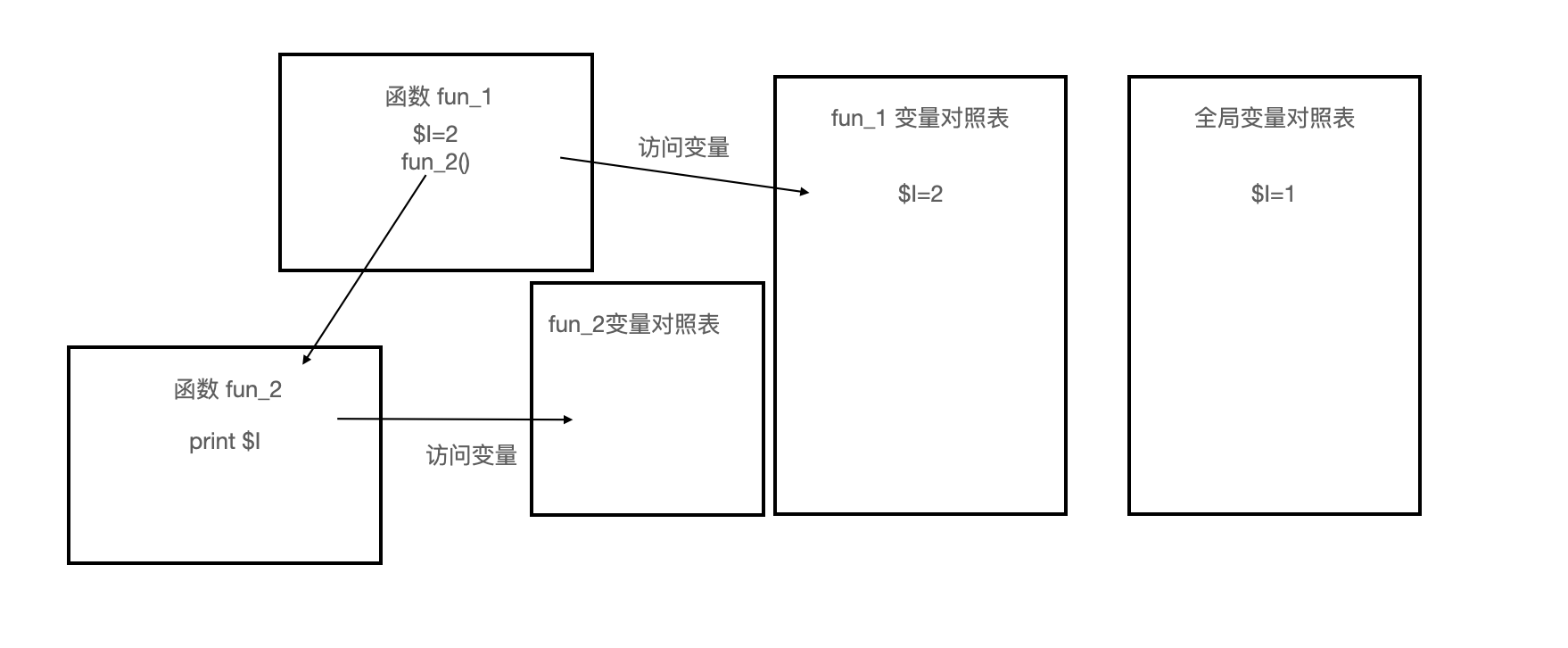### 静态作用域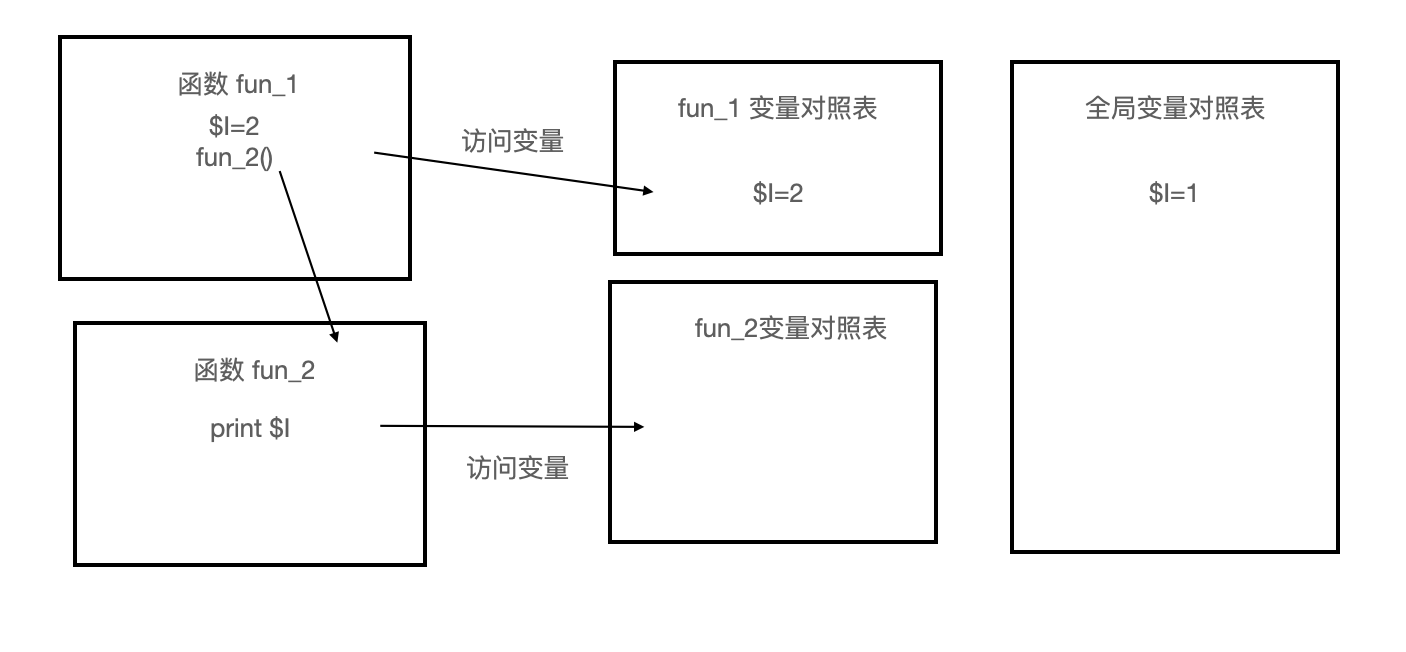# 现象

x = 2

def re_f():
x = 3
def tmp_f(x):
print x
tmp_f(x)

re_f()

• global: 声明全局变量, 既通过静态作用域的方式查找变量
• nolocal: 通过动态作用域的方式查找变量0 评论

0